Articulo

•Citado por SciELO
•Accesos

•Citado por Google
•Similares en SciELO
•Similares en Google

versión impresa ISSN 0012-7353

Dyna rev.fac.nac.minas vol.86 no.208 Medellín ene./mar. 2019

http://dx.doi.org/10.15446/dyna.v86n208.70279

Artículos

Implementation of the ultrasonic through-transmission technique for the elastic characterization of fiber-reinforced laminated composite

Implementación de la técnica de la transmisión ultrasónica para la caracterización de compuestos laminados reforzados con fibras

Carlos Alberto Mezaa  , Ediguer Enrique Francob  , Joao Luis Ealoc  d

a Centro Nacional de Asistencia Técnica a la Industria (ASTIN-SENA), Cali, Colombia. carlos.meva@hotmail.com

c Escuela de Ingeniería Mecánica, Universidad del Valle, Cali, Colombia. joao.ealo@correounivalle.edu.co

d Centro de Investigación e Innovación en Bioinformática y Fotónica - CIBioFi, Universidad del Valle, Cali, Colombia.

Abstract

Laminated composites are widely used in applications when a high strength-to-weight ratio is required. Aeronautic, naval and automotive industries use these materials to reduce the weight of the vehicles and, consequently, fuel consumption. However, the fiber-reinforced laminated materials are anisotropic and the elastic properties can vary widely due to non-standardized manufacturing processes. The elastic characterization using mechanical tests is not easy, destructive and, in most cases, not all the elastic constants can be obtained. Therefore, alternative techniques are required to assure the quality of the mechanical parts and the evaluation of new materials. In this work, the implementation of the ultrasonic through-transmission technique and the characterization of some engineering materials is reported. Isotropic materials and laminated composites of carbon fiber and glass fiber in a polymer matrix were characterized by ultrasound and mechanical tests. An improved methodology for the transit time delay calculation is reported.

Key words: composite material; Christoffel's equation; stiffness tensor; ultrasound; through-transmission technique

Resumen

Los compuestos laminados son usados en aplicaciones donde una alta relación resistencia/peso es requerida. Las industrias aeronáutica, naval y automotriz usan estos materiales para reducir el peso de sus vehículos y ahorrar combustible. Sin embargo, los materiales compuestos laminados son anisotrópicos y sus propiedades varían ampliamente debido a procesos de manufactura no estandarizados. La caracterización elástica de estos materiales usando ensayos mecánicos no es fácil, por lo general las pruebas son destructivas y, en la mayoría de los casos, no es posible obtener todas las constantes elásticas. Por tanto, técnicas de caracterización alternativas son requeridas para asegurar la calidad de las piezas fabricadas y el estudio de nuevos materiales. En este trabajo se reporta la implementación de la técnica de la transmisión ultrasónica y la caracterización de algunos materiales de ingeniería. Muestras de materiales isotrópicos y compuestos laminados de fibra de carbono y vidrio en matriz polimérica fueron caracterizados por ultrasonido y ensayos mecánicos. Se reporta una metodología alternativa para el cálculo de los atrasos.

Palabras-clave: material compuesto; ecuación de Chistoffel; tensor de rigidez; ultrasonido; técnica de la transmisión ultrasónica

1. Introduction

In the most general case, the elastic behavior of an anisotropic material is modeled by 21 independent constants. This number of constants can be reduced, depending on the symmetries in the material structure. For instance, orthotropic materials have different stiffness in three mutually orthogonal directions and are described by 9 independent elastic constants. This is an important group of materials that includes wood and most composite materials. Because of the high anisotropy, the measurement of the stiffness tensor by mechanical tests requires several sample cuts in different directions. However most composites are manufactured or available in sheet form and, in general, it is not possible to have all the required samples .

Ultrasonic non-destructive testing is a widely used technique for inspection and characterization of engineering materials. The behavior of the acoustical waves depends on the elastic properties and the measurement of propagation parameters allows for the characterization of the medium. The main parameter is the wave propagation velocity, directly related to the stiffness. In anisotropic materials, the stiffness and, consequently, the propagation velocity changes depending on the direction.

The first attempts of ultrasonic characterization used transducers in direct contact in order to obtain the propagation velocity in specific directions of high symmetry . This approach allows obtaining only some constants and also requiring samples cut in the specific directions of interest. Another technique uses a fluid coupling, instead of direct contact, in order to propagate bulk waves into the sample. In this case, the sample can be rotated, propagating the waves in many directions. This is called the through-transmission technique, which permits the measurement of the entire stiffness tensor with a single sample .

In the most general case, three waves can coexist inside an anisotropic material: a quasi-longitudinal and two quasi-shear waves, each one with a different propagation speed [4,5]. The mode conversion phenomenon in the fluid-sample interface permits the generation of shear waves into the sample. This is an advantage, because more than one wave can be observed and, consequently, more information of the medium is available. In the case of direct contact, shear waves must be generated using the more expensive and difficult-to-couple shear-wave transducers.

The ultrasonic through-transmission technique is based on the determination of the transit time of the waves into the sample. This is made by comparing the time delay in the cases with and without sample. The case without sample is the reference, where the waves freely propagate from the emitter to the receiver through the coupling fluid. In the case with sample, the material under test is placed between the transducers. If the sample thickness and the incidence angle is known, then a mathematical expression for the propagation velocity in terms of the time delay can be obtained. The Snell's law is required for the calculation of the refraction angles .

The determination of elastic constants of the medium, from a set of bulk ultrasonic wave velocities in an arbitrary direction of the sample, is based on the Christoffel's equation [ 7,5]. Christoffel's equation is a propagation condition of the equation of motion, which establishes the permitted modes of propagation. It is expressed in terms of an eigenvalue-eigenvector problem, where each eigenvalue is related to the propagation velocity of the wave and the respective eigenvector is the propagation direction. In the through-transmission technique, the inverse problem is solved. It means that the propagation speeds are experimentally obtained and the directions are established by the Snell's law. Then, Christoffel's equation is inverted to obtain the elastic tensor.

Despite the experimental simplicity of the technique, there are potential sources of error when measuring velocities. Attention should be paid to parallelism of the sample faces, temperature gradients in the experiment, velocity dispersion, acoustic diffraction, precision in the sample and transducers placement, among others . The high attenuation in composite materials is, probably, the most relevant problem. Besides the low amplitudes obtained in the reception, the strong attenuation at high frequencies distorts the waveform. This phenomenon makes it difficult the comparison of the waves in the calculation of the time delays. Significant errors can be induced as frequency and sample thickness are increased. In addition, there are problems related to the convergence of the inversion algorithm [ 8].

The ultrasonic characterization of anisotropic materials has been studied by many authors in the last years. It can be found the first works in the beginning of the 1970 decade, precisely for ultrasonic characterization of fiber-reinforced composites [9,2]. Before that time, the ultrasonic techniques were mainly applied in the study of crystals. Later, some theoretical works about the wave propagation in materials with triclinic symmetry  and the behavior of the critical angles in composites  were published. However, in the 1990 decade, with the improvement of the electronic instrumentation, the numbers of works had an important increasing. Many works are related to the characterization of the composites employed in the transportation industries [9,8,11-13], the analysis of the technique itself , the characterization of composites reinforced with natural fibers [16 >], ceramic matrix composites  and natural wood . Also, ultrasonic results are used as a basis of comparison in the analysis of other characterization methods .

In this work, the implementation of the ultrasonic through-transmission technique for the characterization of anisotropic materials is reported. A brief revision of the theoretical background is presented. The experimental methodology is described in detail, emphasizing on the time delay calculation, which is a key step in this type of measurement. The main achievement was the recognition of the problems inherent in the conventional methodologies based on the cross correlation and Hilbert's Transformation and the identification of an adequate methodology for the time delay calculation, which leads to accurate elastic constants. The characterization of two isotropic materials of well-known elastic properties was useful for the adjustment of the measurement setup, the signal processing methodology and the algorithm for the inverse problem solution. Samples of laminated composites made of glass and carbon fibers in a polymeric matrix were carefully manufactured. Three samples of each material were characterized by ultrasound and by mechanical tests. The mechanical tests provided a basis of comparison. Results showed a good agreement between ultrasonic and mechanical techniques. Finally, the main advantages of the ultrasonic technique are discussed.

2. Theoretical background

A material with elastic properties that differ in three mutually-orthogonal axes is called orthotropic. In Voigt notation, the stiffness matrix is given by where the number of independent constants is 9 (see Fig. 3 for details of the coordinate system). A special case is a transversely isotropic material, where two of the axes have the same stiffness, forming an isotropic plane. These materials have hexagonal symmetry, with 5 independent constants (C11, C22, C55, C12 y C23). Another special case, the simplest one, is the isotropic material, where the stiffness is the same in the three directions, and the elasticity is modeled by just 2 independent constantsC11 y C12) [20,21]. In the last two cases, the not listed constants are non-independent and they are expressed in terms of the other ones.

The elastic constants are related to wave propagation velocities through the Christoffel's equation [9,13]:

where V is the experimental propagation velocity, ρ is the density and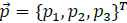is the unit polarization vector. The term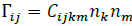is called the Christoffel's tensor, where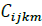is the stiffness tensor, and n k and n m are the components of a vector that points the propagation direction. The Christoffel's equation implements the propagation condition for a plane wave in terms of an eigenvalue/eigenvector problem, where the eigenvalues are related to the propagation velocity and the eigenvectors to the direction. In the case of an orthotropic system, mathematical expressions for Γ 𝑖j , in terms of the components of the stiffness matrix (C ij ) and the direction vector (n 1 ,n 2 and n3), are the following :

Non trivial solutions of the wave equation require that

where δ ij is the Kronecker's delta operator. The symmetry of eq. (4) implies real-valued eigenvalues (pV2) and mutually orthogonal eigenvectors (p k ). Then, three modes can be found for a principal direction, i.e. a longitudinal wave with polarization in the propagation direction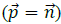and two shear waves with polarization in the orthogonal plane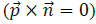.

3. Experimental methods

3.1 Measurement principle

A longitudinal wave with incidence angle θ can excite three different modes inside the sample: a longitudinal wave (α L ) and two shear waves of different velocity (αss stands for the slower wave and α FS for the faster one). These two shear-waves propagate at different speed because the polarization vectors can lay on directions of different stiffness. The experimental procedure entails the determination of the propagation velocity of some, or all, these waves.

Fig. 1 shows a scheme of the measurement principle. Two transducers, an emitter and a receiver, are placed facing each other immersed in a coupling fluid (water in most cases). In the case without sample, the reference wave (α R ) is obtained. When the sample is located between the transducers and rotated an angle θ, the incident wave reach the sample generating the longitudinal (α L ) and the two shear waves (α L and α L ). Due to the different propagation velocities of the waves, they reach the receiver transducer at different time, allowing its identification. The time delay (∆ t ) is calculated by the difference in the time of arrival of each wave with respect to the reference wave. The calculation of ∆ t is an important issue because small errors lead to big inaccuracies in the elastic constants. Three different methods were evaluated and finally one was identified as the most appropriate. An analysis of the ∆ t calculation, comparing the different methodologies, is presented in the results section.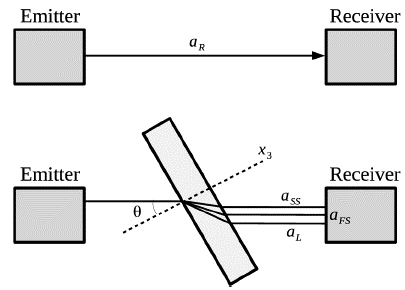Source: The authors.

Figure 1 Measurement principle of the ultrasonic through-transmission technique. α R is a longitudinal reference wave. Three different wave modes are excited: a longitudinal wave (α L ) and two shear waves of different velocity (α ss stands for the slower wave and α FS the faster one).

Propagation velocity is obtained from the experimental ultrasonic signals by 

where V 0 is the reference velocity (sound velocity in the coupling fluid) and h is the sample thickness. The elastic constants are obtained by minimizing the deviation between experimental and theoretical velocities using a least square approach: where n is the number of independent elastic constants, Q is the number of experimental velocities and Vexp q and Vtheo q are the experimental and theoretical velocities, respectively. The theoretical velocities are obtained from an initial value of the stiffness tensor, using the same direction vectors of the experimental case. The determinant of eq. (4) lead to a cubic polynomial in pV2, which can be solved by the Cardano's formula. In some cases, complex eigenvalues are obtained. These unexpected values are certainly consequence of incoherent values of the theoretical elastic constants. It is important to remind that the elastic constants are not completely independent. There are some necessary conditions that must be fulfilled . The least square problem defined in eq. (6) was solved using algorithms for unconstrained and constrained nonlinear optimization.

3.2. Experimental setup

Fig. 2 shows the experimental setup. It is composed by the immersion tank with a mechanical system for the placement of the transducers and the sample rotation. The ultrasonic transducers (Krautkramer MB4S, GE Measurement & Control), with working frequency of 4 MHz, are controlled by an ultrasonic pulser/receiver (5072PR, Olympus NDT, USA). The signals are digitalized in an oscilloscope (TDS2014C, Tektronix, USA) and transferred via USB to a computer for later processing. The computer also controls the sample rotation, using a stepper motor driven by an Arduino board. A specially developed Matlab© script allows the automatic acquisition of the signals.

3.3. Samples

Isotropic and anisotropic samples were characterized in this work. The isotropic samples (AISI 1020 steel and Aluminum alloy A380), with well-known elastic properties, were used to adjust the experimental methodology. The laminated composites were fabricated in the laboratory using unidirectional carbon and glass fiber (one-way patterned fabric) and epoxy resin. All materials were obtained from local suppliers. Density of all samples was obtained by the measurement of the weight and the determination of the volume of the samples geometry. Table 1 shows the properties of the samples, where CFC and GFC refers to the carbon fiber and glass fiber composites, respectively.

Table 1 Physical properties of the samples.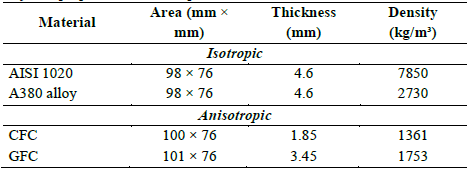Source: The authors.

Fig. 3 shows the coordinate system, where the reinforcing fibers are aligned with the x 1 . axis and the thickness direction with x 3 .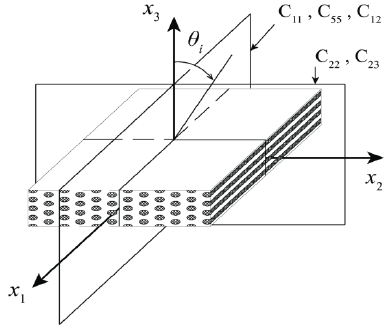Source: The authors.

Figure 3 Sample representation and coordinate system. C ij are independent constants of the stiffness matrix of the sample and θ i is the incidence angle.

4. Results

4.1. Isotropic materials

Fig. 4 shows the experimental and fitted propagation velocities as a function of the incidence angle for the isotropic samples. In the AISI-1020 case (top), a near constant experimental velocity of about 5750 m/s is obtained in the range from normal incidence to 14°. This is the propagation velocity of the longitudinal wave in the steel sample. At 14°, where the velocity suddenly drops, the first critical angle occurs . At this point, the mode conversion is complete, i.e. the transmitted longitudinal wave disappears and only a shear wave remains into the sample. A velocity of 3200 m/s was measured for this wave. Velocity remains constant until 26°, where the second critical angle occurs and the shear waves also disappears. Above 26° all the incident wave is reflected.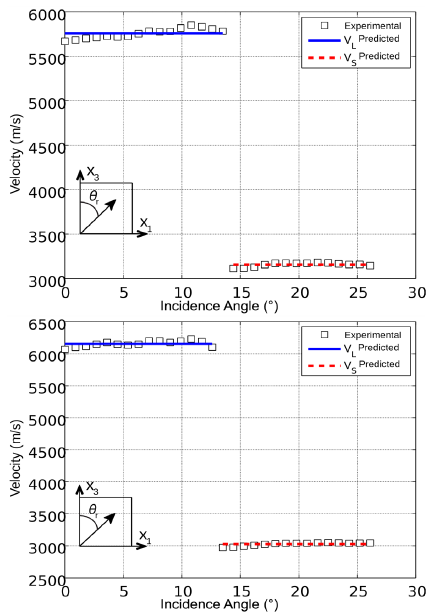Source: The authors.

Figure 4 Wave propagation velocity as a function of the incidence angle for AISI 1020 (top) and aluminum (bottom). V L and V S stand for the longitudinal and shear velocities, respectively.

In the case of the aluminum alloy sample (bottom), the experimental velocities exhibited the same behavior than that of the AISI-1020 sample. The longitudinal and shear velocities were 6150 and 3040 m/s, respectively. The first critical angle is slightly lower (13°). Only one shear-wave is observed because the shear modulus is the same in any direction. Therefore, the propagation velocity is the same regardless of the polarization.

A constant velocity in a range of incidence angles is the expected behavior for isotropic samples. In spite of the possible error sources in the experimental procedure and the delay time calculation, estimated velocity results are in good agreement with the values reported in literature.

The calculation of the elastic constants from the experimental data can be done in different ways. The simplest one is the use of the well-known pair of expressions that relate the longitudinal and shear velocities in an isotropic material to the Young's modulus and the Poisson ratio . Another way is the fitting of the Christoffel's equation to the experimental data, as explained for the anisotropic materials. This second approach was employed in order to test the algorithm for the solution of the inverse problem.

Table 2 shows the elastic constants obtained. Measured and literature values are in agreement. Deviations of less than 2 and 1% for the steel and aluminum alloy samples, respectively, indicate that the measurement methodology implemented proves valid for ultrasonic property extraction of samples.

Table 2 Elastic constants (GPa) of the isotropic materials measured by ultrasound and compared to the literature values. Mean and standard deviation are result of the characterization of three different samples. Deviation is the relative percentage error between literature value and the mean of the experimental one.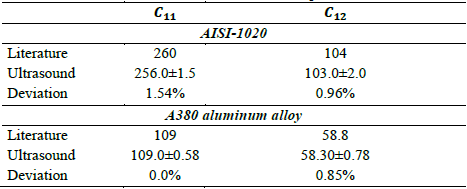Source: The authors.

4.2 Anisotropic materials

Ultrasonic measurements in the fiber reinforced composites were carried out in the isotropic x2-x3 and the anisotropic x1-x3 planes, for incidence angles between 0 and 48 degrees in steps of 1 degree. Theoretically, for each incidence angle there exist three bulk waves generated in the anisotropic sample. However, the shear waves have small amplitude at small angles of incidence and, most times, only the longitudinal wave can be observed. Above certain angle, only shear waves are generated into the sample, but to separate the fast and slow shear waves in the reception could be difficult depending on the sample thickness. In our experiments, only a wave was observed in the range of incidence angles examined.

Fig. 5 shows the results. The isotropic plane of the carbon fiber-polymer composite (top-left) shows a behavior similar to the steel and aluminum alloy samples. The velocities of the longitudinal and shear waves are 2700 and 1380 m/s, respectively. The critical angle occurs at 32°, approximately. It was corroborated that these acoustical properties are close to those of the matrix material, showing the small effect of the reinforcement fibers in these directions.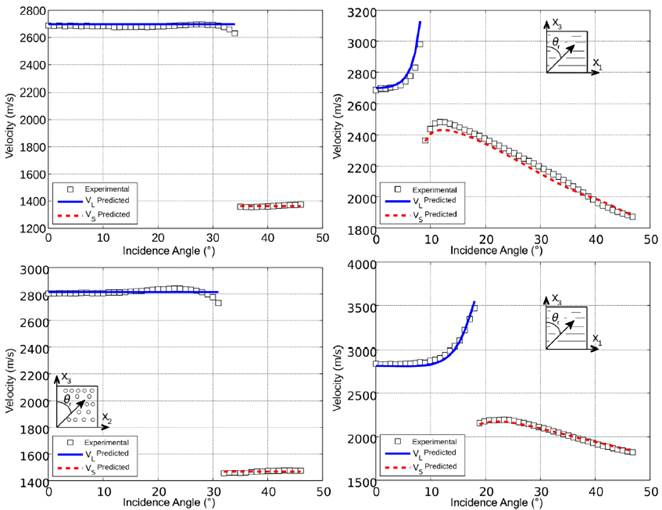Source: The authors.

Figure 5 Propagation velocity as a function of the incidence angle for the laminated composite samples: carbon fiber-polymer (top) and glass fiber-polymer (bottom) composites, for the isotropic x2-x3 (left) and anisotropic x2-x3 (right) planes. VL and VS stand for the longitudinal and shear velocities, respectively.

The anisotropic plane of the carbon fiber-polymer composite (top-right) shows a completely different behavior. A critical angle of about 9° is observed. At normal incidence (θ=0), the velocity of the longitudinal wave is 2700 m/s, the same observed in the isotropic plane. As the incidence angle increases, the wave velocity also increases because the propagation direction gets more and more aligned with the direction of the rigid carbon fibers. Above the critical angle, the shear wave velocity is also dependent on the incidence angle. Just above, the shear velocity is 2380 m/s, increasing up to 2500 m/s at θ=12o. After that, the shear wave velocity monotonously decreases.

Fig. 5 also shows the results of the glass fiber-epoxy composite, for the isotropic (bottom-left) and anisotropic (bottom-right) planes. In general, the behavior is the same observed in the carbon fiber-epoxy composite. The critical angle occurs at 18° and the shear velocity is lower, with a maximum value of 2250 m/s at 22°. These differences are mainly consequence of the properties and mass fraction of the glass fiber. Additionally, the properties of the matrix are also different, surely due to different conditions in the curing process.

Results show a good fitting of the experimental velocities, with a percentage deviation between experimental and fitted value of less than 6% in all cases. Furthermore, the evidence of anisotropy, in addition to an isotropic plane, confirms that the manufactured carbon fiber-epoxy and glass fiber-epoxy composites are transversely isotropic materials.

Table 3 includes the results of the elastic constants obtained by ultrasound. All the components of the stiffness matrix (C ij ) exhibit a low dispersion. To evaluate the validity of the ultrasonic results, mechanical tests were carried out in samples of the same composite materials. Even though, mechanical tests do not allow obtaining all the C ij values. Alternatively, some engineering constants can be determined, e.g. the elastic (E), shear (G) and Poisson (v) moduli . Strain measurements in two directions on the x 1 -x 2 plane allowed us the determination of the, and. Several attempts to obtain E2 with samples cut in the direction perpendicular to the fibers were accomplished. However, because the reinforcement does not do any work in that direction, scattered results (not shown) were obtained.

Table 3 Elastic constants (GPa) of the laminated composites, comparison between the ultrasonic and the mechanical tests. Mean and standard deviation are result of the characterization of three different samples. Deviation is the relative percentage error between the mechanical test value and the mean of the experimental one.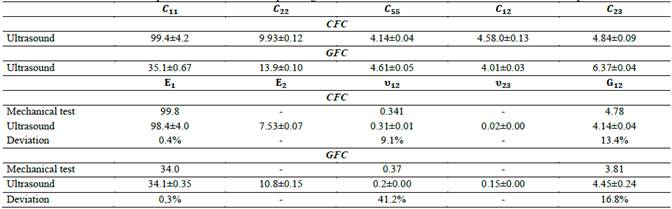Source: The authors.

The results of the elastic modulus in x1 direction (E1) show a good agreement, with relative deviations of 0.3 and 0.4% in the CFC and GFC cases, respectively. In this case, the mechanical load was applied in direction of the reinforcement fibers, which exhibit a more predictable mechanical behavior. For the shear and Poisson moduli, the deviations rise up to 41 and 17%, respectively, where the CFC composite exhibited the better agreement. The high deviation obtained could be consequence of error in the mechanical tests. It is known that the measurements using strain gauges are affected by different factors, such as the coupling of the gauge to the sample, noise in the electronic devices, among others. In conclusion, and considering the variability of the mechanical tests, it is possible to state that the ultrasonic results are sufficiently consistent.

4.3. Determination of the time delay

The calculation of the time delay (∆t) from the acquired ultrasonic signals is an important issue that largely determines the success of the measurement. Three methodologies were tested. In the beginning, two frequently used methodologies based on the cross correlation and the Hilbert transform  were analyzed. The intention was to perform an automated calculation of ∆t, but the complexity of the acoustic signals led to either nonsensical or non-consistent results. To solve the problem, we employed an improved methodology based on the second zero-crossing of the ultrasonic waveform. Fig. 6 shows a comparison of the three methodologies implemented, using the experimental signals obtained with a CFC sample in the anisotropic plane. No step discontinuities were expected as the propagation velocity changes with the angle of incidence, except at the critical angle. However, the methodologies based on the cross correlation exhibit additional discontinuities, leading to big errors in the estimation of the elastic constants. For the type of samples included in this work, this issue was overcome by using the zero-crossing based method, with which the behavior expected is obtained.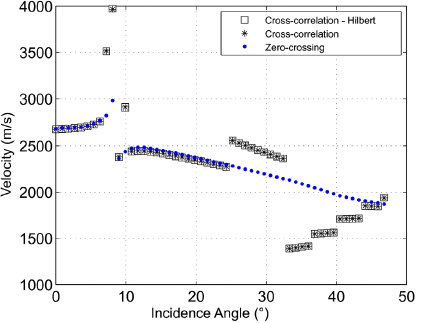Source: The authors.

Figure 6 Velocity as a function of the incidence angle for a CFC sample: results were obtained using the three methodologies used for the determination of the time delay.

Fig. 7 explains the improved method based on a zero-crossing identification. In the reference signal (top), two points are marked out: the first negative peak which exceeds a defined minimum threshold and the subsequent positive peak. Between these two peak amplitudes occurs the zero crossing point to be identified in the waveforms obtained with a given sample. For instance, the signals acquired with a CFC sample at normal (center) and oblique (bottom) incidences are shown. For θ=0, apart from an amplitude decreasing, no significant changes are observed in the waveform when compared to the reference signal. However, for θ=46.8o the distortion becomes evident, i.e. the envelope of the waveform is modified because the maximum amplitude is located at a different position. Because of this, the algorithms based on the cross correlation could fail at oblique incidence. The implemented method includes bounding the signals in a suitable time window, normalization and offset removal (zero mean signals) prior to the Δ𝑡 calculation.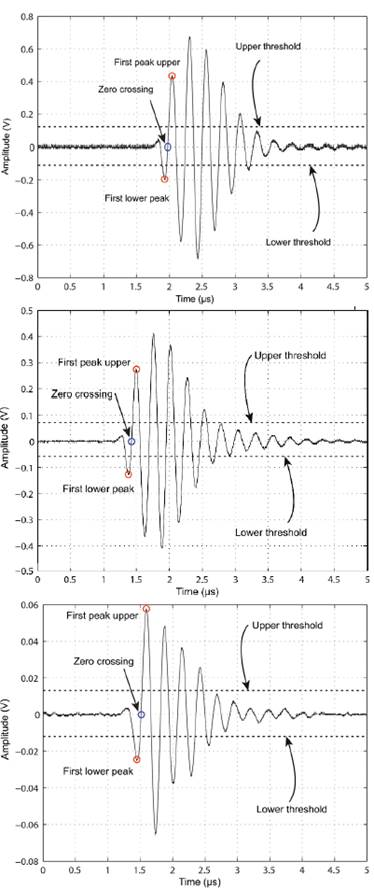Source: The authors.

Figure 7 Zero-crossing based Methodology used in the determination of the time delay for ultrasonic estimation of elastic constant of composite samples. Typical measured waveform. Top: reference signal (no sample between transducers). Center: Signal obtained at normal incidence (θ i =0o). Bottom: Nominal waveform at oblique incidence (θ=46.8o).

5. Conclusions

In this work, the ultrasonic through-transmission technique for the measurement of elastic constants of anisotropic materials was implemented. Theoretical and experimental considerations allowed us to implement a reliable measurement apparatus suitable for the characterization of isotropic and anisotropic samples.

The elastic constants of isotropic samples, steel and aluminum alloy, were extracted with deviations lower than 2% when compared to the values reported in literature. The characterization of these materials of well-known elastic properties was important for the adjustment of the experimental methodology and the solution procedure of the inverse problem involved.

Samples of carbon and glass fiber-reinforced composites were specially manufactured. As the reinforcement is unidirectional, i.e. carbon and glass fiber lay in a single direction; the composites exhibit a transversely isotropic behavior. This was corroborated by the experimental results, where a plane with isotropic behavior was identified in each sample. The other plane clearly exhibited anisotropy, i.e. a dependency of both longitudinal and shear wave velocities on the incidence angle. Further research is deserved to quantify the effect of changes in the fiber-matrix interface region on the ultrasonic extraction of properties using the through-transmission operation mode.

The fitting of the experimental velocity data to the Chistoffel's tensor, by the least square approach proposed, allowed the ultrasonic determination of the elastic constants. The results were compared to those obtained by means of mechanical tests, showing good agreement in the case of E1, with a percentage deviation of less than 0.4% for both, CFC and GFC, samples. The other engineering constants showed higher deviations, but the uncertainty in the shear and Poisson modulus obtained by mechanical test was also considerable. An important advantage of the ultrasonic technique is the ability to provide all elastic constants with a single sample. On contrary, mechanical tests require a set of samples in addition to more expensive equipment and elaborated experimental procedures.

Despite just one wave is observed in the range of incidence angles examined, the complete stiffness tensor can be calculated by performing measurements in different planes. Two planes (x1-x2 and x1-x3) of incidence were sufficient for the determination of the stiffness matrix of the composite materials.

Another important result in this work is the identification of the zero-crossing based method as an improved methodology in the estimation of the ultrasound time delay in the samples under study, in comparison with correlation based methods. Further research is required in order to test the reliability of the proposed methodology for samples with higher degree of anisotropy.

In summary, the ultrasonic through-transmission technique is an efficient and relatively simple technique for the determination of the stiffness matrix of composite materials, with important advantages when compared to mechanical tests.

Acknowledgments

This work was partially supported by the project 110666945414 - UltraVeg (Contrato 567-2014) of the Francisco José De Caldas National Fund for Science, Technology, and Innovation, COLCIENCIAS, Colombia.

References

 Adamowski, J.C., Buiochi, F. and Higuti, R., Ultrasonic material characterization using diffraction-free pvdf receivers. Physics Procedia, 3(1), pp. 593-603, 2010. DOI: 10.1016/j.phpro.2010.01.076 [ Links ]

 Zimmer, J.E. and Cost, J.R., Determination of the elastic constants of a unidirectional fiber composite using ultrasonic velocity measurements. The Journal of the Acoustical Society of America, 47(3), pp. 795-803, 1970. DOI: 10.1121/1.1911962 [ Links ]

 Franco, E.E., Meza, J.M. and Buiochi, F., Measurement of elastic properties of materials by the ultrasonic through-trasnmission technique. Revista Dyna, 78(168), pp. 59-64, 2011. http://www.scielo.org.co/scielo.php?script=sci_arttext&pid=S0012-73532011000400007&lng=en&tlng=enLinks ]

 Sun, M., Optimal recovery of elastic properties for anisotropic materials through ultrasonic measurement. PhD thesis, University of Maine, Orono, Maine, USA, 2002. [ Links ]

 Rose, J.L., Ultrasonic waves in solid media. Pennsylvania: Cambridge University Press, 2004. DOI: 10.1017/CBO9781107273610 [ Links ]

 Rokhlin, S.I. and Wang, W., Double through-transmission bulk wave method for ultrasonic phase velocity measurement and determination of elastic constants of composite materials. The Journal of the Acoustical Society of America, 91(6), pp. 3303-3312, 1992. DOI: 10.1121/1.402847 [ Links ]

 Rokhlin, S., Chimenti, D. and Nag, P., Physical Ultrasonics of Composites. New York: Oxford University Press, 2011. [ Links ]

 Rokhlin, S.I. and Wang, W., Critical angle measurement of elastic constants in composite material. The Journal of the Acoustical Society of America, 86(5), pp. 1876-1882, 1989. DOI: 10.1121/1.398566 [ Links ]

 Markham, M.F., Measurement of the elastic constants of fiber composites by ultrasonics. Composites, 11, pp. 145-149, 1970. DOI: 10.1016/0010-4361(69)90059-7 [ Links ]

 Van Buskirk, W.C., Cowin, S.C. and Carter Jr., R., A theory of acoustic measurement of the elastic constants of a general anisotropic solid. Journal of Materials Science, 21(8), pp. 2759-2762, 1986. DOI: 10.1007/BF00551484 [ Links ]

 Castellano, A., Foti, P., Fraddosio, A., Marzano, S. and Piccioni, M.D., Mechanical characterization of cfrp composites by ultrasonic immersion tests: Experimental and numerical approaches. Composites: Part B, 66(5), pp. 299-310, 2014. DOI: 10.1016/j.compositesb.2014.04.024 [ Links ]

 Aristegui, C. and Baste, S., Optimal recovery of the elasticity tensor of general anisotropic materials from ultrasonic velocity data. The Journal of the Acoustical Society of America, 101(2), pp. 813-833, 1997. DOI: 10.1121/1.418040 [ Links ]

 Balasubramanian, K. and Whitney, S.C., Ultrasonic through-transmission characterization of thick fiber-reinforced composites. NDT & E International, 29(4), pp. 225-236, 1996. DOI: 10.1016/S0963-8695(96)00014-X [ Links ]

 Hosten, B., Stiffness matrix invariants to validate the characterization of composite materials with ultrasonic methods. Ultrasonics, 30(6), pp. 365-370, 1992. DOI: 10.1016/0041-624X(92)90092-Z [ Links ]

 Meza, C.A., Pazos-Ospina, J.F., Franco, E.E., Ealo, J.L., Collazos-Burbano, D.A. and Casanova G.F., Ultrasonic determination of the elastic constants of epoxy-natural fiber composites. Physics Procedia, 70, pp. 467-470, 2015. DOI: 10.1016/j.phpro.2015.08.287 [ Links ]

 Bader, T.K., Dastoorian, F., Ebrahimi, G., Unger, G., Lahayne, O., Hellmich, C. and Pichler, B., Combined ultrasonic-mechanical characterization of orthotropic elastic properties of an unrefined bagasse fiber-polypropylene composite. Composites Part B: Engineering, 95, pp. 96-104, 2016. DOI: 10.1016/j.compositesb.2016.03.070 [ Links ]

 Baste, S. and Morvan, J.M., Under load strain partition of a ceramic matrix composite using an ultrasonic method. Experimental Mechanics, 36(2), pp. 148-154, 1996. DOI: 10.1007/BF02328711 [ Links ]

 Mat, A.N., Jaafar, R., Kadri, S., Hadi, M.I. and Rohani, S., Elastic constant determination of hardwoods using ultrasonic insertion technique. Ultrasonics, 75, pp. 194-198, 2017. DOI: 10.1016/j.ultras.2016.11.025 [ Links ]

 Meza, J.M., Franco, E.E., Farias, M.C., Buiochi, F., Souza, R.M. y Cruz, J., Medición del módulo de elasticidad en materiales de ingeniería utilizando la técnica de indentación instrumentada y de ultrasonido. Revista de Metalurgia, 44(168), pp. 52-65, 2008. DOI: 10.3989/revmetalm.2008.v44.i1.95 [ Links ]

 Bower, A.F. Applied Mechanics of Solids. Boca Raton: CRC Press, 2009. [ Links ]

 Daniel, I.M. and Ishai, O., Engineering Mechanics of Composite Materials. New York: Oxford University Press, 2006. [ Links ]

 Mouhat, F. and Coudert, F.X., Necessary and sufficient elastic stability conditions in various crystal systems. Physical review B, 90 (224104), 2014. DOI: 10.1103/PhysRevB.90.224104 [ Links ]

 Auld, B.A. Acoustic Field and Waves in Solids. New York: John Wiley & Sons, 1973. [ Links ]

How to cite: Meza, C.A., Franco, E.E. and Ealo, J.L., Implementation of the ultrasonic through-transmission technique for the elastic characterization of fiber-reinforced laminated composite.. DYNA, 86(208), pp. 153-161, January - March, 2019

C.A. Meza, received his BSc in Mechanical Engineering in 2012 and his MSc. in Engineering in 2016 of the Universidad del Valle, Colombia, his research was about the use of ultrasonic techniques for the characterization of composite materials, using the technique of ultrasonic transmission. Since 2017 he has worked with SENA, in the area of computer assisted design, CNC machines and additive manufacturing. ORCID: 0000-0002-7999-4583

E.E. Franco, born in Colombia in 1973 and received a BSc. in Mechanical Engineering (2002) from the Universidad de Ibagué at Ibagué, Colombia. He also received a MSc. (2006) and a Dr. degree (2010) from the School of Engineering at the University of São Paulo, Brazil. Since 2010 he is with the Engineering Faculty at Universidad Autónoma de Occidente in Cali, Colombia, supporting teaching and research in the Mechanical Engineering program. The research topics of professor Franco are ultrasonic characterization of materials, development of piezoelectric devices, mechanical vibrations and numerical methods. ORCID: 0000-0001-7518-704X

J.L. Ealo, is BSc. in Mechanical Engineering in 1998 and an MSc. in Industrial Control Systems in 2000. In 2009 he obtained a Dr. degree in Mechanical Engineering (Technical University of Madrid-UPM, Madrid, Spain) for which his dissertation dealt with the design of ferroelectret based transducers for ultrasonic applications in air. Since 2002 he is with the School of Mechanical Engineering of the Universidad del Valle, in Santiago de Cali, Colombia. In 2011 he founded the Laboratory in Vibrations and Acoustics (LaVA) to support teaching and research, at undergraduate and graduate levels, in dynamics of systems and machinery, acoustics and mechanics of materials, ultrasonic non-destructive testing, transducer design, physics of musical instruments and physical acoustics. ORCID: 0000-0002-9708-7178

Recibido: 07 de Febrero de 2018; Revisado: 12 de Noviembre de 2018; Aprobado: 10 de Diciembre de 2018This is an open-access article distributed under the terms of the Creative Commons Attribution License